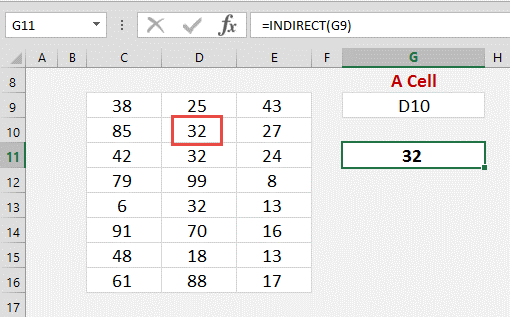# The Importance Of INDIRECT FunctionINDIRECT Function yields a reference to a range. The range being referred can be a named range, a range of cells or can be a cell. The formula is represented as

=INDIRECT(ref_text, [a1])

Several excel users have been mystified with the usage of INDIRECT function. If you are of the opinion that this function does not have a lot to offer to the users, then think again!  There are several ways in which this function can be used in a workbook.

• Referenced range is a cell

The content of the referenced cell is returned when the referenced range is a cell. For instance, if in cell G9 you enter D10 in A1 style and in a different cell you enter INDIRECT (G9), then this returns the value of the cell D10. From the following example, 32 is the number which is returned indirectly.1. Reference is a range of cells

The INDIRECT function returns the contents of the cells referenced, when the referenced range considered is a range of cells. The syntax is represented as

=SUM(INDIRECT(C9:E9))

The SUM function can then be entered which totals the referenced cells. For instance, if in cell I9, C9:E9 is entered in A1 style and in a different cell you enter INDIRECT (I9), then this will sum the values indirectly present within the cells. In the following example, 106 is the value returned.

1. Reference is a named range

2. The INDIRECT function returns the content of named range when the referenced range is a named range. A sum function can be entered that will sum up the named ranged. A named range needs to be created by entering a name in the Name Box as well as by choosing the data range. The syntax is:  =SUM(INDIRECT(NamedRange)).

3. Consider having several Named ranges within a Workbook that refer different data sets. This technique can be employed for choosing several data sets and summation of each can be obtained by using the INDIRECT function.